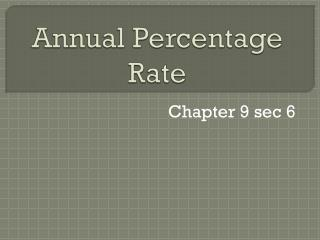DownloadDownload PresentationAnnual Percentage Rate

# Annual Percentage Rate

Télécharger la présentation## Annual Percentage Rate

- - - - - - - - - - - - - - - - - - - - - - - - - - - E N D - - - - - - - - - - - - - - - - - - - - - - - - - - -
##### Presentation Transcript

1. Annual Percentage Rate Chapter 9 sec 6

2. Questions • How many of you have bought a car?, House?, furniture?, RV?, boat?,… • What made you decide to buy these things? • Did you look for anything special? • For example, any special deals, bargains?

3. Problem Lets say you bought a couch for \$3000(don’t forget the interest) in 3 yearly payments using an add-on interest rate of 10%. What is your true interest rate?

4. APR What is the “true” interest rate? And what does it mean? The “true” interest rate is the called the annual percentage rate, or APR, which we will denote by a.

5. Year 1 Using the add-on from sec 9.2 we can compute the I = Prt= (3000)(.10)(3) = \$900. Therefore the amount to be repaid in 3 equal installments is 3000 + 900 = 3900. Divide by 3, \$1300 of which \$1000 is being paid by the principle and \$300 is the interest. Solving the equation; 300 = (3000)(r)(1)= for r and r = .10. Therefore your interest is 10%.

6. Year 2 At the end of the second year, you make another payment of \$1300, of which \$1000 goes to reduce the principle and \$300 is interest. For the 2nd year you have paid \$300 interest for \$2000 loan. Solving the equation 300 = (2000)(r)(1) = .15. Therefore in reality, the interest rate on your loan for the second year is 15%.

7. Final year, Year 3 Sorry, it gets worst for you!! You make a final payment of \$1300. Paid \$1000 on the remaining principle and \$300 for the interest. Solving the equation 300 = (1000)(r)(1) = .30 Now the interest rate is 30%

8. You got robbed!!!!!! You better be pissed!!!!

9. BUT HOLD ON!!!!

10. Lets look back at the Problem. The interest for the first year + interest for the second year + interest for the third year = \$900. Using I = Prt, the equation will look like; (3000)(a)(1) + (2000)(a)(1) + (1000)(a)(1) = 900

11. Solve for a, 6000a = 900 a = 0.15. Therefore the APR is 15%. You can check it if you borrow \$3000 for 1 year at 15% and then \$2000 for 1 year at 15% and then \$1000 or 1 year at 15%, the total interest for the 3 years is \$900.

12. Finding the APR

13. Using the Table 1. Find the finance charge on the loan if it is not already given to you. 2. Determine the finance charge per \$100 on the loan. 3. use the line of Table that corresponds to the number of payments to find the number closest to the amount found in step 2. 4. The top of the column containing the number found is step 3 is the APR.

14. Example In order to use the table, you must first know the finance charge on the loan. If you borrow \$780 and pay a finance charge of \$148.20, then the finance charge per \$100 of the amount is given by FC is the finance charge.

15. Problem Ann has agreed to pay off a \$5000 loan by making 12 monthly payments. If the total finance charge on his loan is \$410, what is the APR she is being charged?

16. Ann is making 12 monthly payments. Using the table. The closest amount is \$8.31, therefore it is approximate APR for her loan is 15%.

17. Buying a Car. Nate is considering buying a car costing \$18,580. The terms of the sale require a down payment of \$3200 and the rest to be paid off by making 36 monthly payments of \$310 each. What is the APR will she be paying on the car financing?

18. Amount being finance is the purchase price minus the down payment, which is \$18,580 - \$3200 = \$ 15,380. Because her payment amount is 36 x 310 = \$11,160, this makes the finance charge equal to \$15,380 - \$11,160 = \$4220. Using the table the finance charge is per \$100.

19. Now look at the row of 36 payments. The APR for this car is approximately a little over 16%.

20. Therefore knowing your APR is important.